Observing points on the graph

Chapter 3 Class 9 Coordinate Geometry
Concept wise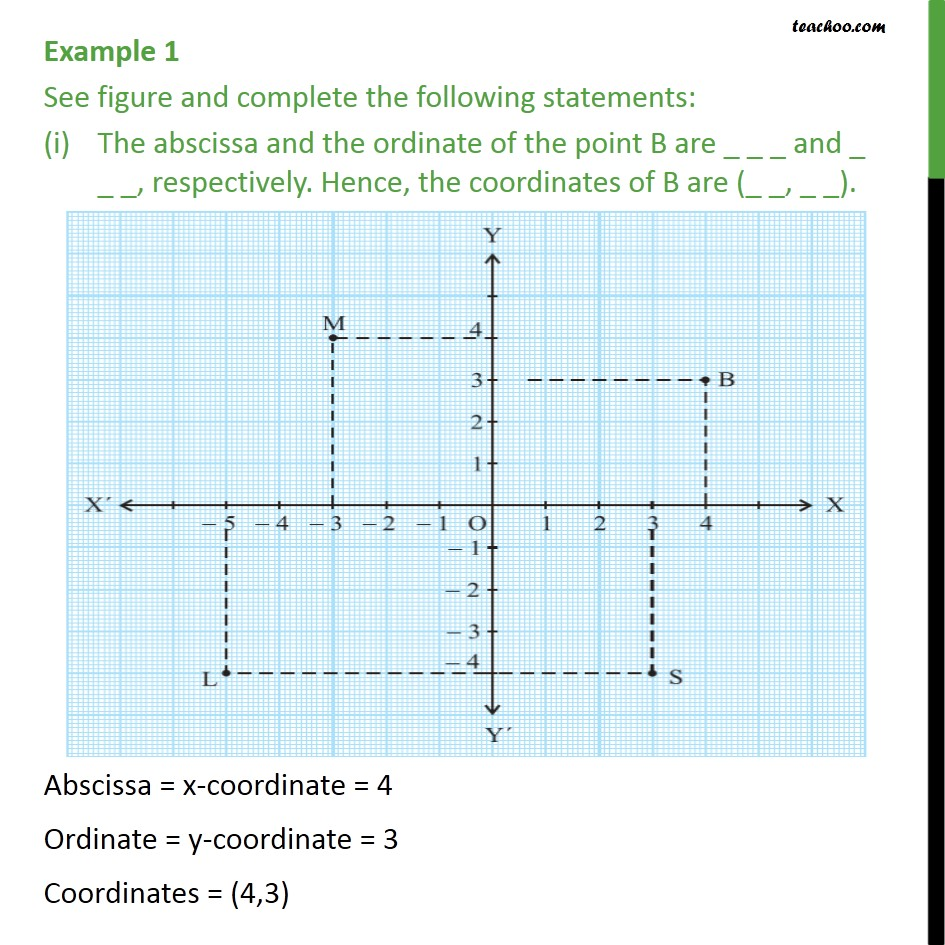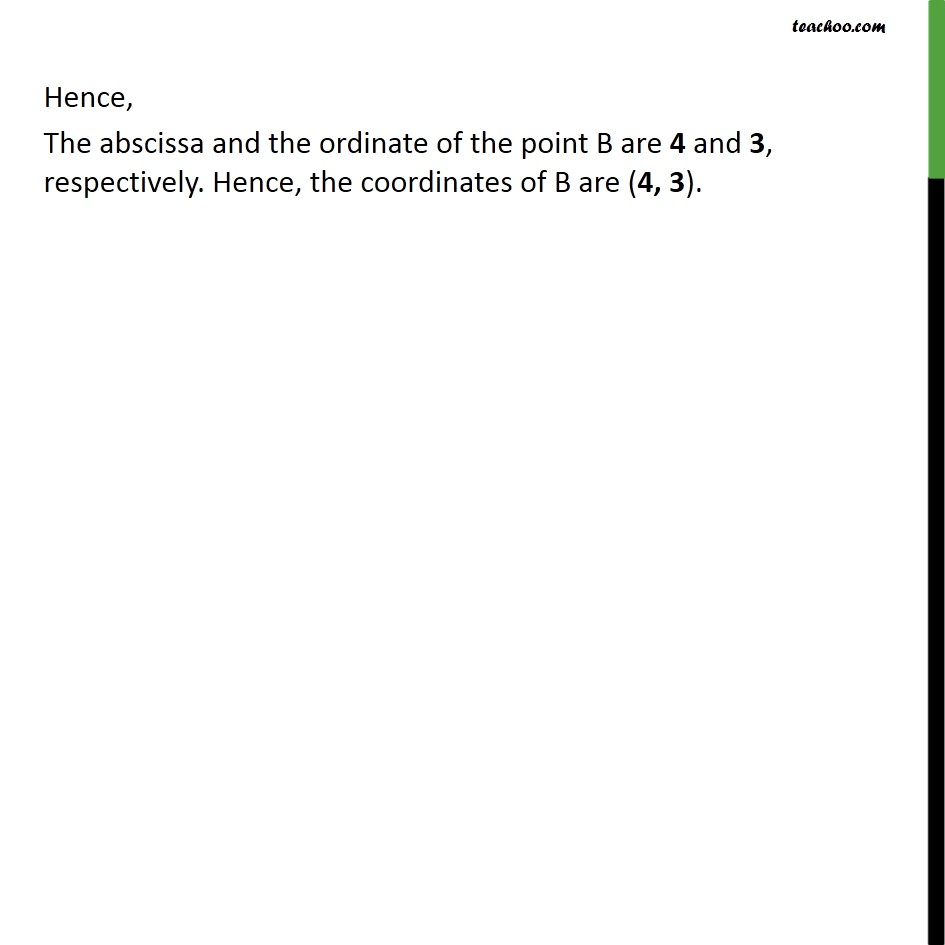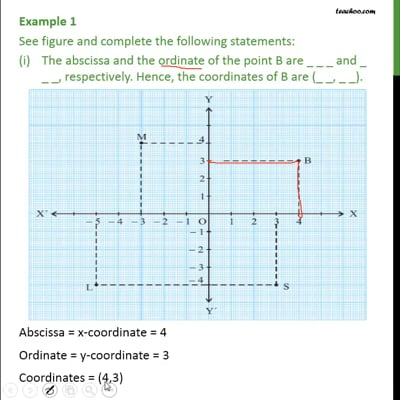This video is only available for Teachoo black users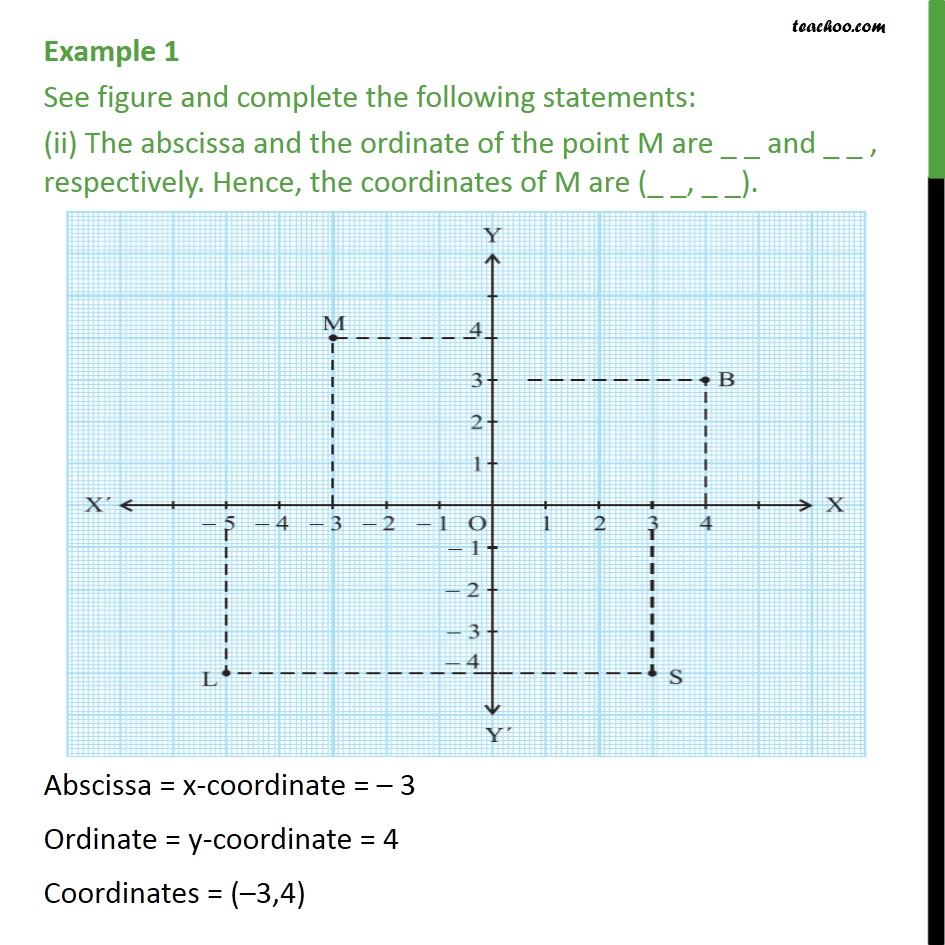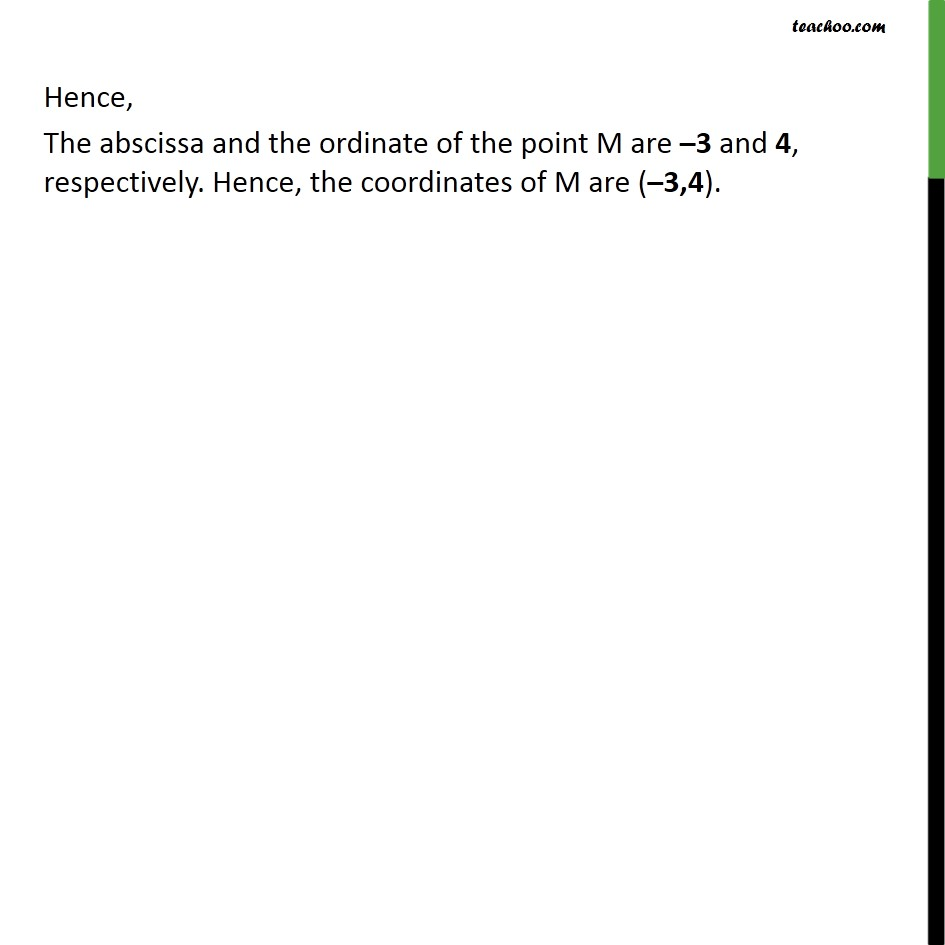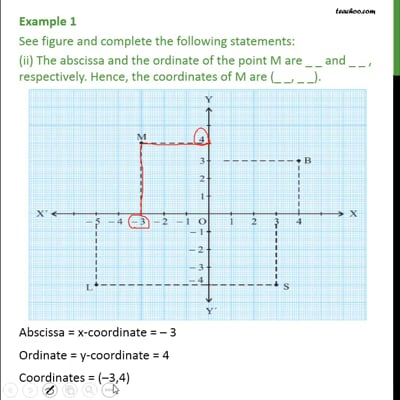This video is only available for Teachoo black users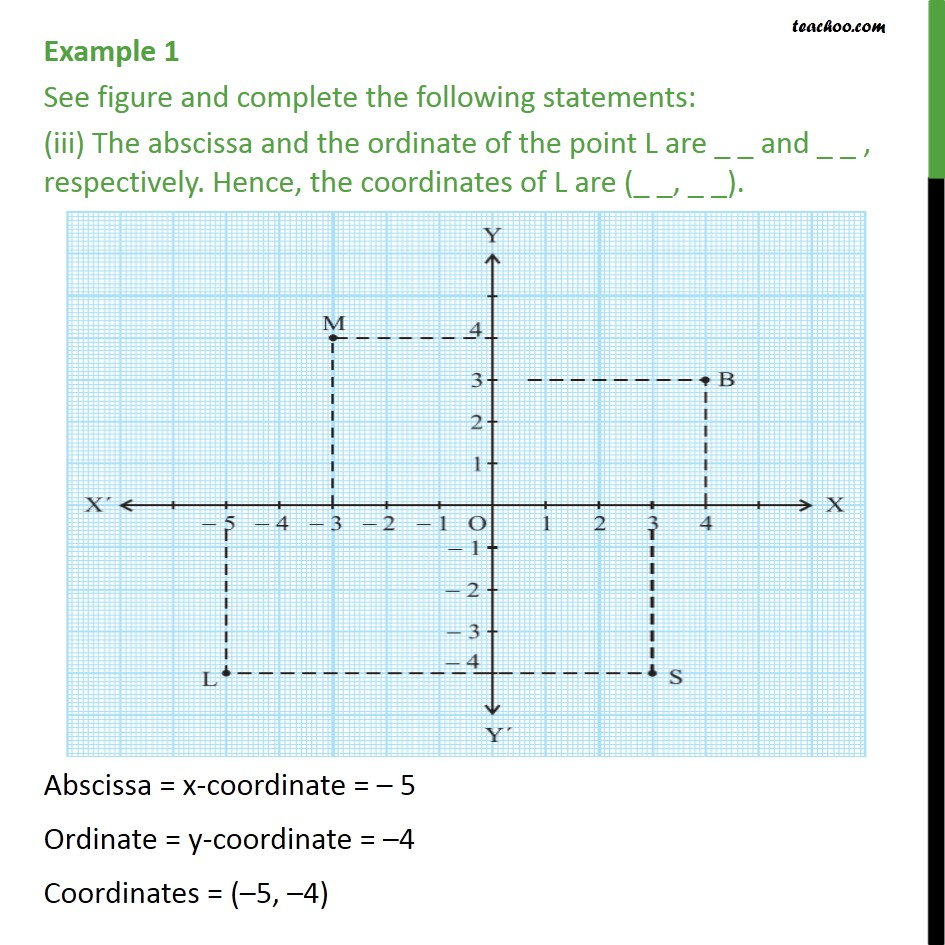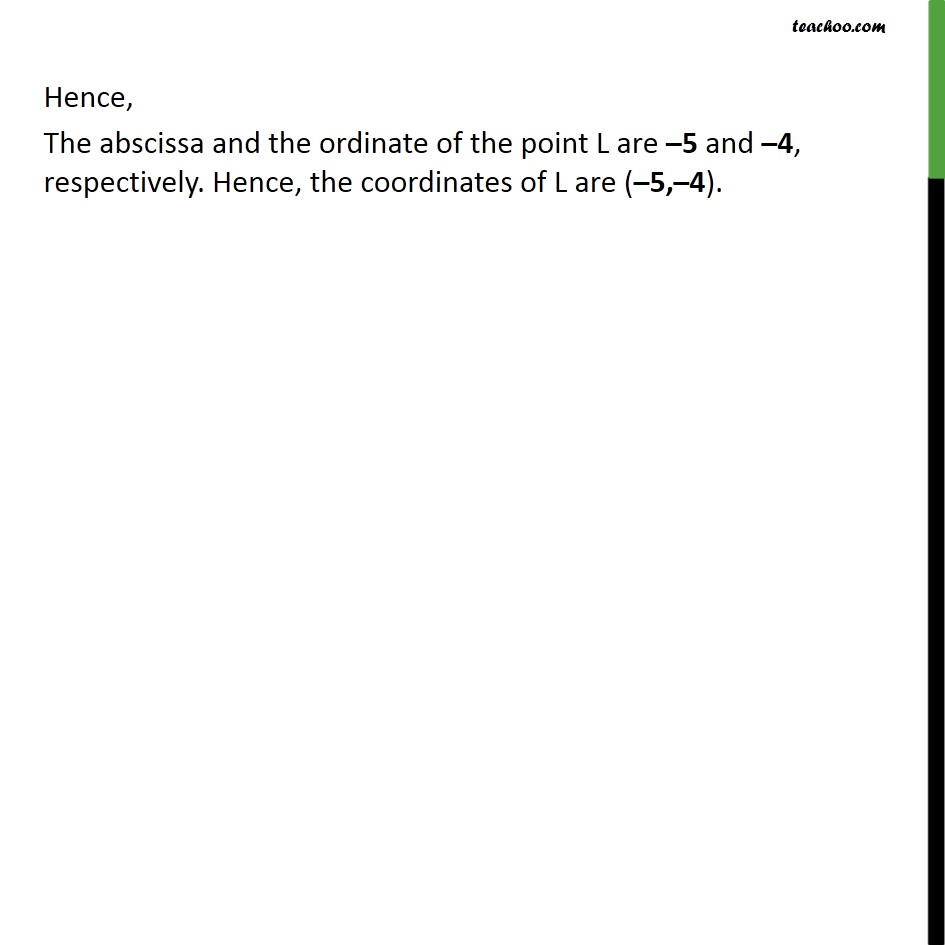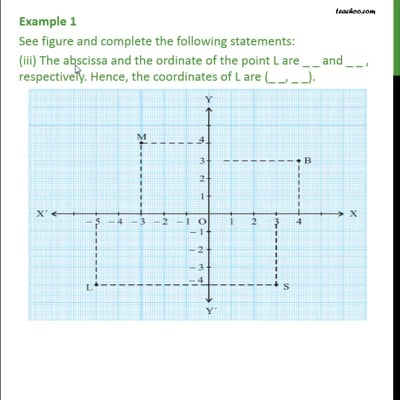This video is only available for Teachoo black users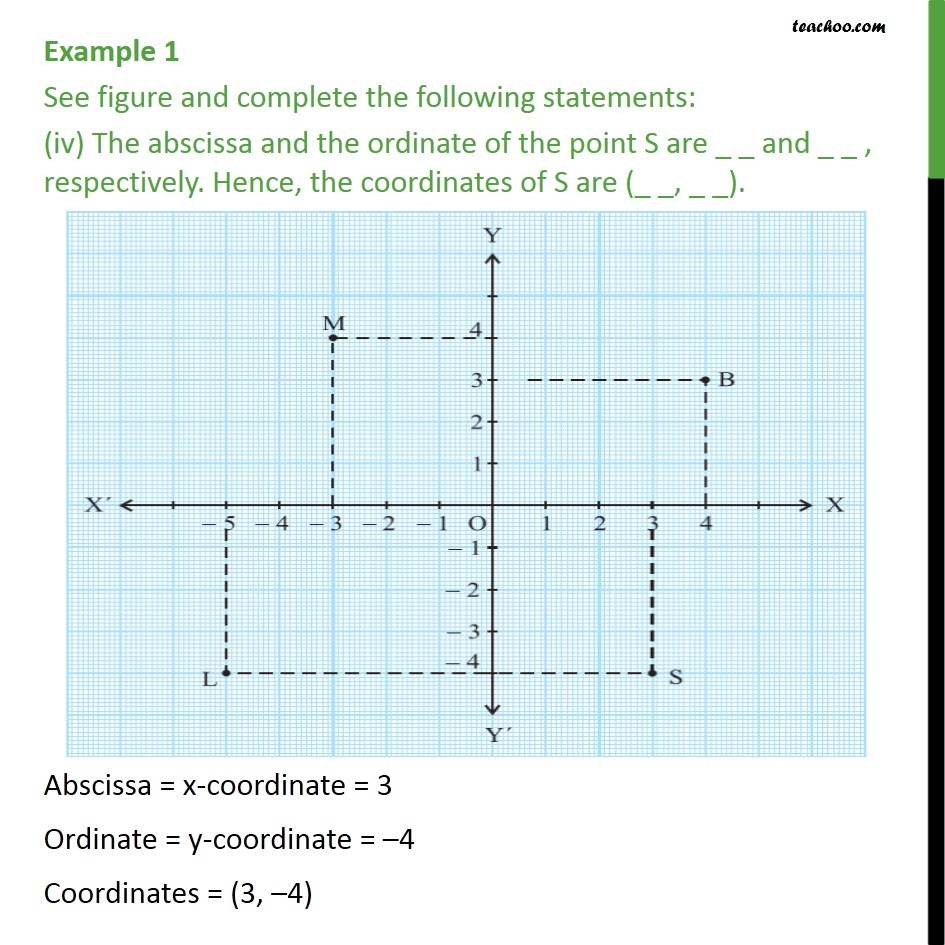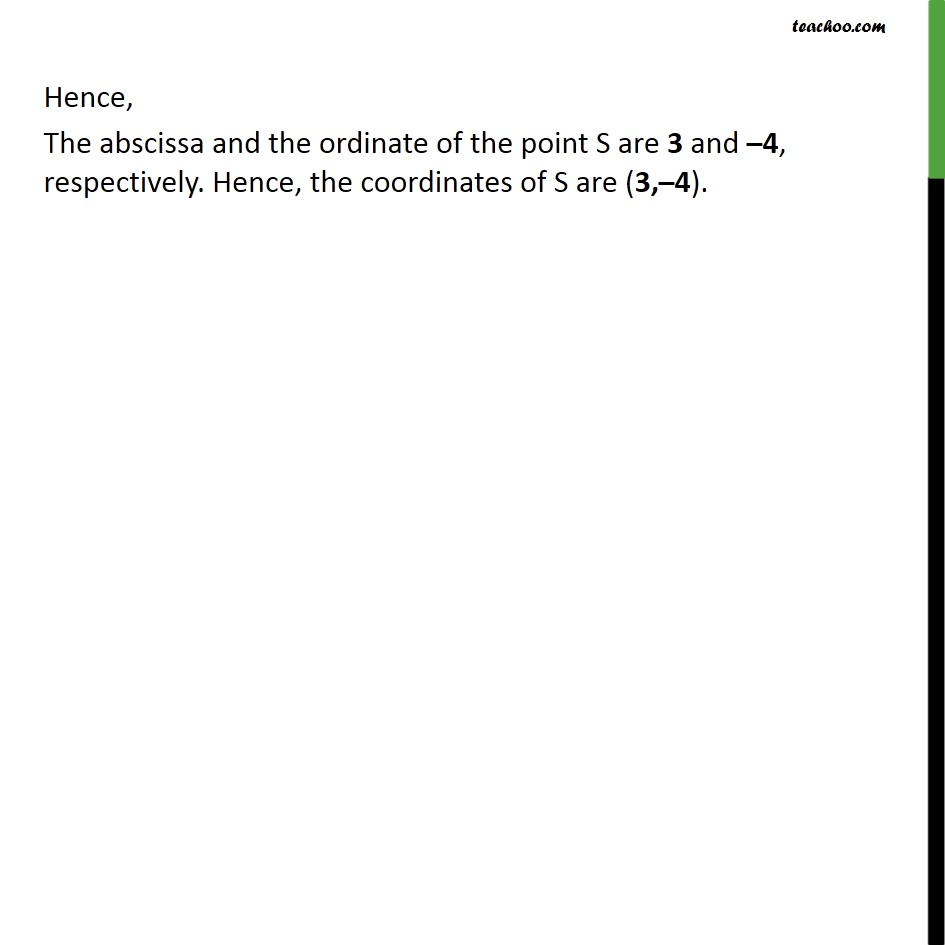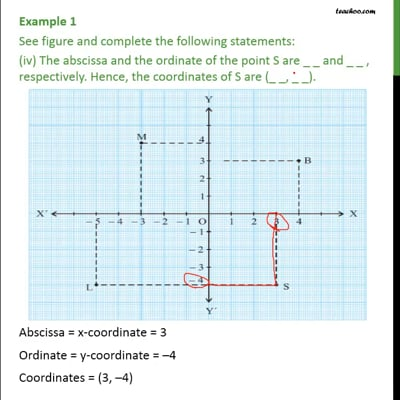This video is only available for Teachoo black users

Introducing your new favourite teacher - Teachoo Black, at only ₹83 per month

### Transcript

Example 1 See figure and complete the following statements: The abscissa and the ordinate of the point B are _ _ _ and _ _ _, respectively. Hence, the coordinates of B are (_ _, _ _). Abscissa = x-coordinate = 4 Ordinate = y-coordinate = 3 Coordinates = (4,3) Hence, The abscissa and the ordinate of the point B are 4 and 3, respectively. Hence, the coordinates of B are (4, 3). Example 1 See figure and complete the following statements: (ii) The abscissa and the ordinate of the point M are _ _ and _ _ , respectively. Hence, the coordinates of M are (_ _, _ _). Abscissa = x-coordinate = – 3 Ordinate = y-coordinate = 4 Coordinates = (–3,4) Hence, The abscissa and the ordinate of the point M are –3 and 4, respectively. Hence, the coordinates of M are (–3,4). Example 1 See figure and complete the following statements: (iii) The abscissa and the ordinate of the point L are _ _ and _ _ , respectively. Hence, the coordinates of L are (_ _, _ _). Abscissa = x-coordinate = – 5 Ordinate = y-coordinate = –4 Coordinates = (–5, –4) Hence, The abscissa and the ordinate of the point L are –5 and –4, respectively. Hence, the coordinates of L are (–5,–4). Example 1 See figure and complete the following statements: (iv) The abscissa and the ordinate of the point S are _ _ and _ _ , respectively. Hence, the coordinates of S are (_ _, _ _). Abscissa = x-coordinate = 3 Ordinate = y-coordinate = –4 Coordinates = (3, –4) Hence, The abscissa and the ordinate of the point S are 3 and –4, respectively. Hence, the coordinates of S are (3,–4).